#### unmerged(25633)

##### First Lieutenant
Given that I don't know of any military role played by Poniatowski...

And, if there was no better Polish commander allied with the Swedes than Sapieha, then perhaps that Leader ought to be eliminated althogether.

It's not like the Swedes are short of leaders after all--especially if Rehnskiold's stats are improved to better reflect historical reality

The Corsair

#### unmerged(80927)

##### Corporal
Code:
``````#-----------------------------------------------------------------------------------------------
#XVI century

id = { type = 6 id = 0131804 }
category = general
startdate = {
year = 1506
}
deathdate = {
day = 15
month = december
year = 1520
}
rank = 3
movement = 2
fire = 2
shock = 2
siege = 1
dormant = no
}
id = { type = 6 id = 0131807 }
category = general
name = "Berend von Mehlen"
startdate = {
year = 1521
}
deathdate = {
year = 1525
}
rank = 1
movement = 2
fire = 2
shock = 3
siege = 0
dormant = no
}
id = { type = 6 id = 0131808 }
category = general
name = "Johan Tre Rosor"
startdate = {
year = 1534
}
deathdate = {
year = 1554
}
rank = 1
movement = 3
fire = 2
shock = 3
siege = 0
dormant = no
}
id = { type = 6 id = 0131805 }
name = "Jakob Bagge"
startdate = {
year = 1555
}
deathdate = {
year = 1570
}
rank = 1
movement = 4
fire = 3
shock = 3
siege = 0
dormant = no
}
id = { type = 6 id = 0131811 }
category = general
name = "Nils Boije" #Nils Boije af Gennäs
startdate = {
day = 26
month = october
year = 1555
}
deathdate = {
month = february
year = 1566
}
rank = 2
movement = 2
fire = 2
shock = 2
siege = 1
dormant = no
remark = "Took the strong castle of Varberg in 1565, but defeated while trying to take the castle of Bohus."
}
id = { type = 6 id = 0131813 }
category = general
name = "Charles de Mornay"
startdate = {
year = 1563
}
deathdate = {
year = 1570
}
rank = 3
movement = 3
fire = 3
shock = 3
siege = 0
dormant = no
}
id = { type = 6 id = 0131814 }
category = general
name = "Henrik Horn" #Henrik Horn Klasson af Kancas
startdate = {
month = october
year = 1563
}
deathdate = {
year = 1584
}
rank = 2
movement = 3
fire = 3
shock = 3
siege = 0
dormant = no
remark = "Son of Klas Horn."
}
id = { type = 6 id = 0131810 }
name = "Klas Horn"
startdate = {
day = 7
month = august
year = 1564
}
deathdate = {
month = september
year = 1566
}
rank = 1
movement = 4
fire = 5
shock = 5
siege = 0
dormant = no
}
id = { type = 6 id = 0131816 }
category = general
name = "Karl"
startdate = {
year = 1569
}
deathdate = {
day = 20
month = december
year = 1605
}
rank = 1
movement = 3
fire = 2
shock = 2
siege = 0
dormant = no
remark = "Karl IX before reign" #Hertig Karl
}
id = { type = 6 id = 0131817 }
category = general
name = "Karl Horn"
startdate = {
year = 1577
}
deathdate = {
day = 15
month = may
year = 1601
}
rank = 1
movement = 3
fire = 4
shock = 3
siege = 1
dormant = no
remark = "Son of Henrik Horn. Both were tireless in Estland."
}
id = { type = 6 id = 0131815 }
category = general
name = "P. de la Gardie"
startdate = {
year = 1580
}
deathdate = {
day = 4
month = november
year = 1585
}
rank = 1
movement = 4
fire = 3
shock = 3
siege = 1
dormant = no
}
id = { type = 6 id = 0131809 }
category = general
name = "Klas E. Fleming"
startdate = {
year = 1591
}
deathdate = {
day = 11
month = april
year = 1597
}
rank = 1
movement = 3
fire = 3
shock = 3
siege = 0
dormant = no
}

#-----------------------------------------------------------------------------------------------
#XVII century

id = { type = 6 id = 0131818 }
category = general
name = "Carl Gyllenhielm"
startdate = {
year = 1601
}
deathdate = {
year = 1611
}
rank = 2
movement = 3
fire = 2
shock = 3
siege = 0
dormant = no
remark = "Bastard Brother of Gustav II Adolf"
}

id = { type = 6 id = 0131820 }
category = general
name = "J. de la Gardie"
startdate = {
year = 1608
}
deathdate = {
year = 1628
}
rank = 1
movement = 2
fire = 3
shock = 3
siege = 0
dormant = no
remark = "Laiska-Jaakko"
}
id = { type = 6 id = 0131821 }
category = general
name = "Evert Horn"
startdate = {
year = 1609
}
deathdate = {
day = 29
month = july
year = 1615
}
rank = 3
movement = 3
fire = 4
shock = 3
siege = 2
dormant = no
remark = "Son of Karl Horn and daring friend of J.De La Gardie. One of few Swedish siege experts."
}
id = { type = 6 id = 0131822 }
name = "Klas Fleming"
startdate = {
year = 1619
}
deathdate = {
day = 25
month = july
year = 1644
}
rank = 1
movement = 3
fire = 3
shock = 3
siege = 0
dormant = no
}
id = { type = 6 id = 0131819 }
category = general
name = "Herman Wrangel"
startdate = {
year = 1626
}
deathdate = {
year = 1637
}
rank = 3
movement = 3
fire = 4
shock = 3
siege = 0
dormant = no
}
id = { type = 6 id = 0131824 }
category = general
name = "Gustav Horn"
startdate = {
year = 1628
}
deathdate = {
day = 9
month = may
year = 1657
}
rank = 1
movement = 4
fire = 3
shock = 3
siege = 0
dormant = no
}
id = { type = 6 id = 0131827 }
category = general
name = "Bernhard"
startdate = {
year = 1631
}
deathdate = {
day = 4
month = september
year = 1635
}
rank = 1
movement = 3
fire = 4
shock = 4
siege = 1
dormant = no
remark = "...of Saxe-Weimar. Actually became a French general in 1635."
}
id = { type = 6 id = 0131851 }
category = general
name = "Torten Stålhandske"
startdate = {
year = 1632
}
deathdate = {
day = 20
month = april
year = 1644
}
rank = 2
movement = 3
fire = 2
shock = 4
siege = 0
dormant = no
}
id = { type = 6 id = 0131825 }
category = general
name = "Johan Banér"
startdate = {
year = 1634
}
deathdate = {
day = 9
month = may
year = 1641
}
rank = 1
movement = 5
fire = 4
shock = 5
siege = 1
dormant = no
}
id = { type = 6 id = 0131828 }
category = general
name = "Karl G. Wrangel" #Karl Gustav Wrangel
startdate = {
year = 1638
}
deathdate = {
day = 18
month = june
year = 1675
}
rank = 1
movement = 4
fire = 4
shock = 3
siege = 1
dormant = no
}
id = { type = 6 id = 0131829 }
category = general
name = "Arvid Wittenberg"
startdate = {
day = 9
month = may
year = 1641
}
deathdate = {
day = 6
month = september
year = 1657
}
rank = 1
movement = 4
fire = 4
shock = 3
siege = 0
dormant = no
}
id = { type = 6 id = 0131826 }
category = general
name = "Torstenson"
startdate = {
day = 30
month = august
year = 1641
}
deathdate = {
month = december
year = 1646
}
rank = 1
movement = 5
fire = 5
shock = 4
siege = 1
dormant = no
}
id = { type = 6 id = 0131830 }
category = general
name = "von Königsmarck" #Hans Christoffer
startdate = {
year = 1642
}
deathdate = {
year = 1655
}
rank = 1
movement = 5
fire = 3
shock = 4
siege = 0
dormant = no
remark = "Commander of the Flying Column"
}
id = { type = 6 id = 0131853 }
category = general
name = "G.A Lewenhaupt" #Gustaf Adolf Lewenhaupt
startdate = {
year = 1645
}
deathdate = {
day = 28
month = november
year = 1656
}
rank = 1
movement = 4
fire = 3
shock = 3
siege = 1
dormant = no
remark = "Took Daugavpils. Organized the defense of Finland just before his death"
}
id = { type = 6 id = 0131833 }
category = monarch
name = "Karl X Gustav"
startdate = {
year = 1648
}
deathdate = {
day = 12
month = february
year = 1660
}
rank = 0
movement = 5
fire = 4
shock = 4
siege = 1
dormant = no
remark = "King"
}
id = { type = 6 id = 0131832 }
category = general
name = "R. von Ascheberg"
startdate = {
year = 1656
}
deathdate = {
year = 1681
}
rank = 1
movement = 4
fire = 3
shock = 4
siege = 0
dormant = no
remark = "Rutger von Ascheberg, a very able commander."
}
id = { type = 6 id = 0131835 }
name = "Gustav Stenbock"
startdate = {
year = 1664
}
deathdate = {
year = 1676
}
rank = 1
movement = 3
fire = 3
shock = 3
siege = 0
dormant = no
}
id = { type = 6 id = 0131834 }
category = monarch
name = "Karl XI"
startdate = {
month = april
year = 1676
}
deathdate = {
day = 4
month = april
year = 1697
}
rank = 0
movement = 5
fire = 3
shock = 3
siege = 0
dormant = no
remark = "King"
}
id = { type = 6 id = 0131842 }
name = "Hans Wachtmeister"
startdate = {
month = june
year = 1676
}
deathdate = {
day = 14
month = february
year = 1714
}
rank = 1
movement = 4
fire = 3
shock = 3
siege = 0
dormant = no
}
id = { type = 6 id = 0131837 }
category = general
name = "C.G Rehnskiöld" #Carl Gustav
startdate = {
year = 1699
}
deathdate = {
day = 28
month = january
year = 1722
}
rank = 1
movement = 3
fire = 3
shock = 4
siege = 0
dormant = no
}

#-----------------------------------------------------------------------------------------------
#XVIII century

id = { type = 6 id = 0131843 }
category = general
name = "A.L Lewenhaupt" #Adam Ludwig
startdate = {
year = 1701
}
deathdate = {
day = 11
month = february
year = 1719
}
rank = 1
movement = 2
fire = 3
shock = 4
siege = 0
dormant = no
}
id = { type = 6 id = 0131841 }
category = general
name = "Magnus Stenbock"
startdate = {
year = 1704
}
deathdate = {
day = 22
month = february
year = 1717
}
rank = 1
movement = 3
fire = 4
shock = 4
siege = 0
dormant = no
}
id = { type = 6 id = 0131836 }
category = general
name = "Carl G. Armfeldt" #Carl Gustav
startdate = {
day = 5
month = august
year = 1713
}
deathdate = {
day = 23
month = october
year = 1736
}
rank = 2
movement = 3
fire = 3
shock = 3
siege = 0
dormant = no
}
id = { type = 6 id = 0131846 }
category = general
name = "Axel von Fersen"
startdate = {
year = 1748
}
deathdate = {
year = 1765
}
rank = 3
movement = 3
fire = 3
shock = 3
siege = 0
dormant = no
}
id = { type = 6 id = 0131845 }
category = general
name = "A. Ehrensvärd" #Augustin
startdate = {
year = 1756
}
deathdate = {
day = 28
month = july
year = 1771
}
rank = 1
movement = 3
fire = 4
shock = 3
siege = 1
dormant = no
remark = "The man behind Sveaborg. Also an admiral."
}
id = { type = 6 id = 0131848 }
name = "Henrik af Trolle"
startdate = {
year = 1773
}
deathdate = {
day = 11
month = march
year = 1784
}
rank = 1
movement = 4
fire = 3
shock = 4
siege = 0
dormant = no
}

#-----------------------------------------------------------------------------------------------
#XIX century

id = { type = 6 id = 0131850 }
category = general
startdate = {
year = 1808
}
deathdate = {
day = 20
month = august
year = 1815
}
rank = 1
movement = 3
fire = 3
shock = 3
siege = 0
dormant = no
remark = "See the battles of Revolahti (Revolax) and Oravainen (Oravais)"
}
id = { type = 6 id = 0131849 }
category = general
name = "Carl von Döbeln" #Georg Carl von Döbeln
startdate = {
year = 1808
}
deathdate = {
day = 15
month = february
year = 1820
}
rank = 2
movement = 4
fire = 3
shock = 4
siege = 0
dormant = no
remark = "Battle of Kauhajoki. Also fought in India in French service."
}
id = { type = 6 id = 0131852 }
category = general
name = "Johan A. Sandels" #Johan August Sandels
startdate = {
year = 1808
}
deathdate = {
year = 1827
}
rank = 2
movement = 5
fire = 3
shock = 4
siege = 0
dormant = no
remark = "Battle of Koljonvirta (Virta bro)"
}

#-----------------------------------------------------------------------------------------------

#First free id: 0131859
#Ids 0131806, 0131812, 0131839, 0131844 and 0131838 are also free``````

Gathenhielm, Arvid Horn, Lars Eriksson and Bo Grip have been removed and Toll replaced and everyone else has been reworked, more or less. Gathenhielm was removed because he was a privateer and pirate and didn't often follow the crown's orders and because he didn't command any naval battles. I have found absolutely zero information on this "Lars Eriksson". Removed until somebody finds some something on him. Bo Grip's only defense was that of his castle, and not terribly successfully, so he's been removed as well. This "Hans Boije af Gennäs" was actually Nils Boije af Gennäs (an ancestor of mine) so he's been changed.

I've also added a new leader, a Lewenhaupt, to replace Toll and asked a Polish historian, Radosław Sikora, regarding Sapieha and Poniatowski. I think Poniatowski should be removed, unless Mr Sikora manages to dig up some actually competent Polish commander in Karl XII's service.

Also I think Sweden might have too many leaders during the middle of the 17th century, but we'll see.

EDIT: Gustav Adolf and Karl XII removed, since I didn't do anything for them
EDIT2: Poniatowski removed.

Last edited:

#### Toio

##### Field Marshal
•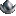•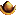•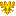•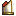••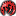Code:
``````#-----------------------------------------------------------------------------------------------
#XVI century

id = { type = 6 id = 0131804 }
category = general
startdate = {
year = 1506
}
deathdate = {
day = 15
month = december
year = 1520
}
rank = 3
movement = 2
fire = 2
shock = 2
siege = 1
dormant = no
}
id = { type = 6 id = 0131807 }
category = general
name = "Berend von Mehlen"
startdate = {
year = 1521
}
deathdate = {
year = 1525
}
rank = 1
movement = 2
fire = 2
shock = 3
siege = 0
dormant = no
}
id = { type = 6 id = 0131808 }
category = general
name = "Johan Tre Rosor"
startdate = {
year = 1534
}
deathdate = {
year = 1554
}
rank = 1
movement = 3
fire = 2
shock = 3
siege = 0
dormant = no
}
id = { type = 6 id = 0131805 }
name = "Jakob Bagge"
startdate = {
year = 1555
}
deathdate = {
year = 1570
}
rank = 1
movement = 4
fire = 3
shock = 3
siege = 0
dormant = no
}
id = { type = 6 id = 0131811 }
category = general
name = "Nils Boije" #Nils Boije af Gennäs
startdate = {
day = 26
month = october
year = 1555
}
deathdate = {
month = february
year = 1566
}
rank = 2
movement = 2
fire = 2
shock = 2
siege = 1
dormant = no
remark = "Took the strong castle of Varberg in 1565, but defeated while trying to take the castle of Bohus."
}
id = { type = 6 id = 0131813 }
category = general
name = "Charles de Mornay"
startdate = {
year = 1563
}
deathdate = {
year = 1570
}
rank = 3
movement = 3
fire = 3
shock = 3
siege = 0
dormant = no
}
id = { type = 6 id = 0131814 }
category = general
name = "Henrik Horn" #Henrik Horn Klasson af Kancas
startdate = {
month = october
year = 1563
}
deathdate = {
year = 1584
}
rank = 2
movement = 3
fire = 3
shock = 3
siege = 0
dormant = no
remark = "Son of Klas Horn."
}
id = { type = 6 id = 0131810 }
name = "Klas Horn"
startdate = {
day = 7
month = august
year = 1564
}
deathdate = {
month = september
year = 1566
}
rank = 1
movement = 4
fire = 5
shock = 5
siege = 0
dormant = no
}
id = { type = 6 id = 0131816 }
category = general
name = "Karl"
startdate = {
year = 1569
}
deathdate = {
day = 20
month = december
year = 1605
}
rank = 1
movement = 3
fire = 2
shock = 2
siege = 0
dormant = no
remark = "Karl IX before reign" #Hertig Karl
}
id = { type = 6 id = 0131817 }
category = general
name = "Karl Horn"
startdate = {
year = 1577
}
deathdate = {
day = 15
month = may
year = 1601
}
rank = 1
movement = 3
fire = 4
shock = 3
siege = 1
dormant = no
remark = "Son of Henrik Horn. Both were tireless in Estland."
}
id = { type = 6 id = 0131815 }
category = general
name = "P. de la Gardie"
startdate = {
year = 1580
}
deathdate = {
day = 4
month = november
year = 1585
}
rank = 1
movement = 4
fire = 3
shock = 3
siege = 1
dormant = no
}
id = { type = 6 id = 0131809 }
category = general
name = "Klas E. Fleming"
startdate = {
year = 1591
}
deathdate = {
day = 11
month = april
year = 1597
}
rank = 1
movement = 3
fire = 3
shock = 3
siege = 0
dormant = no
}

#-----------------------------------------------------------------------------------------------
#XVII century

id = { type = 6 id = 0131818 }
category = general
name = "Carl Gyllenhielm"
startdate = {
year = 1601
}
deathdate = {
year = 1611
}
rank = 2
movement = 3
fire = 2
shock = 3
siege = 0
dormant = no
remark = "Bastard Brother of Gustav II Adolf"
}

id = { type = 6 id = 0131820 }
category = general
name = "J. de la Gardie"
startdate = {
year = 1608
}
deathdate = {
year = 1628
}
rank = 1
movement = 2
fire = 3
shock = 3
siege = 0
dormant = no
remark = "Laiska-Jaakko"
}
id = { type = 6 id = 0131821 }
category = general
name = "Evert Horn"
startdate = {
year = 1609
}
deathdate = {
day = 29
month = july
year = 1615
}
rank = 3
movement = 3
fire = 4
shock = 3
siege = 2
dormant = no
remark = "Son of Karl Horn and daring friend of J.De La Gardie. One of few Swedish siege experts."
}
id = { type = 6 id = 0131822 }
name = "Klas Fleming"
startdate = {
year = 1619
}
deathdate = {
day = 25
month = july
year = 1644
}
rank = 1
movement = 3
fire = 3
shock = 3
siege = 0
dormant = no
}
id = { type = 6 id = 0131819 }
category = general
name = "Herman Wrangel"
startdate = {
year = 1626
}
deathdate = {
year = 1637
}
rank = 3
movement = 3
fire = 4
shock = 3
siege = 0
dormant = no
}
id = { type = 6 id = 0131824 }
category = general
name = "Gustav Horn"
startdate = {
year = 1628
}
deathdate = {
day = 9
month = may
year = 1657
}
rank = 1
movement = 4
fire = 3
shock = 3
siege = 0
dormant = no
}
id = { type = 6 id = 0131827 }
category = general
name = "Bernhard"
startdate = {
year = 1631
}
deathdate = {
day = 4
month = september
year = 1635
}
rank = 1
movement = 3
fire = 4
shock = 4
siege = 1
dormant = no
remark = "...of Saxe-Weimar. Actually became a French general in 1635."
}
id = { type = 6 id = 0131851 }
category = general
name = "Torten Stålhandske"
startdate = {
year = 1632
}
deathdate = {
day = 20
month = april
year = 1644
}
rank = 2
movement = 3
fire = 2
shock = 4
siege = 0
dormant = no
}
id = { type = 6 id = 0131825 }
category = general
name = "Johan Banér"
startdate = {
year = 1634
}
deathdate = {
day = 9
month = may
year = 1641
}
rank = 1
movement = 5
fire = 4
shock = 5
siege = 1
dormant = no
}
id = { type = 6 id = 0131828 }
category = general
name = "Karl G. Wrangel" #Karl Gustav Wrangel
startdate = {
year = 1638
}
deathdate = {
day = 18
month = june
year = 1675
}
rank = 1
movement = 4
fire = 4
shock = 3
siege = 1
dormant = no
}
id = { type = 6 id = 0131829 }
category = general
name = "Arvid Wittenberg"
startdate = {
day = 9
month = may
year = 1641
}
deathdate = {
day = 6
month = september
year = 1657
}
rank = 1
movement = 4
fire = 4
shock = 3
siege = 0
dormant = no
}
id = { type = 6 id = 0131826 }
category = general
name = "Torstenson"
startdate = {
day = 30
month = august
year = 1641
}
deathdate = {
month = december
year = 1646
}
rank = 1
movement = 5
fire = 5
shock = 4
siege = 1
dormant = no
}
id = { type = 6 id = 0131830 }
category = general
name = "von Königsmarck" #Hans Christoffer
startdate = {
year = 1642
}
deathdate = {
year = 1655
}
rank = 1
movement = 5
fire = 3
shock = 4
siege = 0
dormant = no
remark = "Commander of the Flying Column"
}
id = { type = 6 id = 0131853 }
category = general
name = "G.A Lewenhaupt" #Gustaf Adolf Lewenhaupt
startdate = {
year = 1645
}
deathdate = {
day = 28
month = november
year = 1656
}
rank = 1
movement = 4
fire = 3
shock = 3
siege = 1
dormant = no
remark = "Took Daugavpils. Organized the defense of Finland just before his death"
}
id = { type = 6 id = 0131833 }
category = monarch
name = "Karl X Gustav"
startdate = {
year = 1648
}
deathdate = {
day = 12
month = february
year = 1660
}
rank = 0
movement = 5
fire = 4
shock = 4
siege = 1
dormant = no
remark = "King"
}
id = { type = 6 id = 0131832 }
category = general
name = "R. von Ascheberg"
startdate = {
year = 1656
}
deathdate = {
year = 1681
}
rank = 1
movement = 4
fire = 3
shock = 4
siege = 0
dormant = no
remark = "Rutger von Ascheberg, a very able commander."
}
id = { type = 6 id = 0131835 }
name = "Gustav Stenbock"
startdate = {
year = 1664
}
deathdate = {
year = 1676
}
rank = 1
movement = 3
fire = 3
shock = 3
siege = 0
dormant = no
}
id = { type = 6 id = 0131834 }
category = monarch
name = "Karl XI"
startdate = {
month = april
year = 1676
}
deathdate = {
day = 4
month = april
year = 1697
}
rank = 0
movement = 5
fire = 3
shock = 3
siege = 0
dormant = no
remark = "King"
}
id = { type = 6 id = 0131842 }
name = "Hans Wachtmeister"
startdate = {
month = june
year = 1676
}
deathdate = {
day = 14
month = february
year = 1714
}
rank = 1
movement = 4
fire = 3
shock = 3
siege = 0
dormant = no
}
id = { type = 6 id = 0131837 }
category = general
name = "C.G Rehnskiöld" #Carl Gustav
startdate = {
year = 1699
}
deathdate = {
day = 28
month = january
year = 1722
}
rank = 1
movement = 3
fire = 3
shock = 4
siege = 0
dormant = no
}

#-----------------------------------------------------------------------------------------------
#XVIII century

id = { type = 6 id = 0131843 }
category = general
name = "A.L Lewenhaupt" #Adam Ludwig
startdate = {
year = 1701
}
deathdate = {
day = 11
month = february
year = 1719
}
rank = 1
movement = 2
fire = 3
shock = 4
siege = 0
dormant = no
}
id = { type = 6 id = 0131839 }
category = general
name = "S. Poniatowski"
startdate = {
year = 1702
}
deathdate = {
year = 1714
}
rank = 3
movement = 2
fire = 2
shock = 2
siege = 0
dormant = no
remark = "Also Polish."
}
id = { type = 6 id = 0131841 }
category = general
name = "Magnus Stenbock"
startdate = {
year = 1704
}
deathdate = {
day = 22
month = february
year = 1717
}
rank = 1
movement = 3
fire = 4
shock = 4
siege = 0
dormant = no
}
id = { type = 6 id = 0131836 }
category = general
name = "Carl G. Armfeldt" #Carl Gustav
startdate = {
day = 5
month = august
year = 1713
}
deathdate = {
day = 23
month = october
year = 1736
}
rank = 2
movement = 3
fire = 3
shock = 3
siege = 0
dormant = no
}
id = { type = 6 id = 0131846 }
category = general
name = "Axel von Fersen"
startdate = {
year = 1748
}
deathdate = {
year = 1765
}
rank = 3
movement = 3
fire = 3
shock = 3
siege = 0
dormant = no
}
id = { type = 6 id = 0131845 }
category = general
name = "A. Ehrensvärd" #Augustin
startdate = {
year = 1756
}
deathdate = {
day = 28
month = july
year = 1771
}
rank = 1
movement = 3
fire = 4
shock = 3
siege = 1
dormant = no
remark = "The man behind Sveaborg. Also an admiral."
}
id = { type = 6 id = 0131848 }
name = "Henrik af Trolle"
startdate = {
year = 1773
}
deathdate = {
day = 11
month = march
year = 1784
}
rank = 1
movement = 4
fire = 3
shock = 4
siege = 0
dormant = no
}

#-----------------------------------------------------------------------------------------------
#XIX century

id = { type = 6 id = 0131850 }
category = general
startdate = {
year = 1808
}
deathdate = {
day = 20
month = august
year = 1815
}
rank = 1
movement = 3
fire = 3
shock = 3
siege = 0
dormant = no
remark = "See the battles of Revolahti (Revolax) and Oravainen (Oravais)"
}
id = { type = 6 id = 0131849 }
category = general
name = "Carl von Döbeln" #Georg Carl von Döbeln
startdate = {
year = 1808
}
deathdate = {
day = 15
month = february
year = 1820
}
rank = 2
movement = 4
fire = 3
shock = 4
siege = 0
dormant = no
remark = "Battle of Kauhajoki. Also fought in India in French service."
}
id = { type = 6 id = 0131852 }
category = general
name = "Johan A. Sandels" #Johan August Sandels
startdate = {
year = 1808
}
deathdate = {
year = 1827
}
rank = 2
movement = 5
fire = 3
shock = 4
siege = 0
dormant = no
remark = "Battle of Koljonvirta (Virta bro)"
}

#-----------------------------------------------------------------------------------------------

#First free id: 0131859
#Ids 0131806 0131812 0131844 0131838 are also free``````

Gathenhielm, Arvid Horn, Lars Eriksson and Bo Grip have been removed and Toll replaced and everyone else has been reworked, more or less. Gathenhielm was removed because he was a privateer and pirate and didn't often follow the crown's orders and because he didn't command any naval battles. I have found absolutely zero information on this "Lars Eriksson". Removed until somebody finds some something on him. Bo Grip's only defense was that of his castle, and not terribly successfully, so he's been removed as well. This "Hans Boije af Gennäs" was actually Nils Boije af Gennäs (an ancestor of mine) so he's been changed.

I've also added a new leader, a Lewenhaupt, to replace Toll and asked a Polish historian, Radosław Sikora, regarding Sapieha and Poniatowski. I think Poniatowski should be removed, unless Mr Sikora manages to dig up some actually competent Polish commander in Karl XII's service.

Also I think Sweden might have too many leaders during the middle of the 17th century, but we'll see.

EDIT: Gustav Adolf and Karl XII removed, since I didn't do anything for them

i also think SWE has too many leaders in that period, so which from your list are new or need to be removed, can you please colour code them, ie red for out , yellow for in

As regards for kings, they should be left out as leaders unless they personally led their armies.

#### unmerged(80927)

##### Corporal
Code:
``````[COLOR="Red"]historicalleader = {
id = { type = 6 id = 0131819 }
category = general
name = "Herman Wrangel"
startdate = {
year = 1626
}
deathdate = {
year = 1637
}
rank = 3
movement = 3
fire = 4
shock = 3
siege = 0
dormant = no
}
id = { type = 6 id = 0131851 }
category = general
name = "Torsten Stålhandske"
startdate = {
year = 1632
}
deathdate = {
day = 20
month = april
year = 1644
}
rank = 2
movement = 3
fire = 2
shock = 4
siege = 0
dormant = no
}
id = { type = 6 id = 0131830 }
category = general
name = "von Königsmarck" #Hans Christoffer
startdate = {
year = 1642
}
deathdate = {
year = 1655
}
rank = 1
movement = 5
fire = 3
shock = 4
siege = 0
dormant = no
remark = "Commander of the Flying Column"
}
id = { type = 6 id = 0131853 }
category = general
name = "G.A Lewenhaupt" #Gustaf Adolf Lewenhaupt
startdate = {
year = 1645
}
deathdate = {
day = 28
month = november
year = 1656
}
rank = 1
movement = 4
fire = 3
shock = 3
siege = 1
dormant = no
remark = "Took Daugavpils. Organized the defense of Finland just before his death"
}
id = { type = 6 id = 0131832 }
category = general
name = "R. von Ascheberg"
startdate = {
year = 1656
}
deathdate = {
year = 1681
}
rank = 1
movement = 4
fire = 3
shock = 4
siege = 0
dormant = no
remark = "Rutger von Ascheberg, a very able commander."
}
id = { type = 6 id = 0131835 }
category = general
name = "Gustav Stenbock"
startdate = {
year = 1642
}
deathdate = {
year = 1664
}
rank = 1
movement = 3
fire = 3
shock = 3
siege = 0
dormant = no
}
[/COLOR]
#-----------------------------------------------------------------------------------------------

#First free id: 0131859
#Ids 0131806, 0131812, [COLOR="Yellow"]0131819, 0131832[/COLOR] ,0131838 and 0131844 are also free``````

Herman Wrangel and Rutger von Ascheberg should be removed since they were fairly insignificant and the others marked with red can be removed if necessary. I've also changed Gustav Stenbock into a general, because while his rank was an admiral he actually commanded only land troops. He can be removed as well, if necessary.

As regards for kings, they should be left out as leaders unless they personally led their armies.

And ? Sweden at the moment has Karl Knutsson Bonde, Sten Sture den äldre, Gustav II Adolf, Karl X, Karl XI, Karl XII and Karl Johan XIV who were de facto rulers of Sweden during their time as a leader in game. None of the should be removed, since all of them commanded campaigns personally. I only meant I removed Gustav II Adolf and Karl XII from the list because I didn't do anything for them and including them in my list would therefore be useless. I didn't mean they should be removed from the game.

#### unmerged(80927)

##### Corporal
Ok, so according to Radek the best Polish commanders were Hieronim Augustyn Lubomirski and Michał Serwacy Wiśniowiecki. However both of these were in the service of Karl's puppet king of the Commonwealth, rather than Karl himself. So I don't think they qualify. Removed, unless someone disagrees.

EDIT: Actually, Lubomirski wasn't even in the service of Stanisław Leszczyński but only of the Warsaw confederation...

Last edited:

#### unmerged(25633)

##### First Lieutenant
Legu wrote:
Herman Wrangel and Rutger von Ascheberg should be removed since they were fairly insignificant and the others marked with red can be removed if necessary. I've also changed Gustav Stenbock into a general, because while his rank was an admiral he actually commanded only land troops. He can be removed as well, if necessary.

corsair:
In my homegrown, I use Simon Grundel-Helmfeldt instead of Stenbock.

From the rest of your list above, I use only Konigsmarck and von Ascheberg (with drastically reduced dates)

el ausente

#### unmerged(80927)

##### Corporal
I've done some tweaking here and there and unless someone disagrees with my changes I'll submit this one as per the rules.

Code:
``````#Sweden - Leaders

#-----------------------------------------------------------------------------------------------
#XV century

id = { type = 6 id = 0131800 }
category = general
name = "Engelbrekt"
startdate = {
day = 10
month = january
year = 1434
}
deathdate = {
day = 4
month = may
year = 1436
}
rank = 5
movement = 2
fire = 2
shock = 2
siege = 1
dormant = no
}
id = { type = 6 id = 0131801 }
category = monarch
name = "Karl Knutsson" #Karl Knutsson Bonde
startdate = {
year = 1436
}
deathdate = {
day = 24
month = february
year = 1457
}
rank = 0
movement = 3
fire = 2
shock = 2
siege = 0
dormant = no
remark = "Karl VIII"
}
id = { type = 6 id = 0131802 }
category = general
name = "Sten Sture d.ä"	#Sten Sture den äldre (the elder)
startdate = {
year = 1470
}
deathdate = {
year = 1497
}
rank = 2
movement = 3
fire = 3
shock = 3
siege = 0
dormant = no
}
id = { type = 6 id = 0131803 }
category = general
name = "Knut Posse"
startdate = {
year = 1471
}
deathdate = {
day = 24
month = march
year = 1500
}
rank = 2
movement = 2
fire = 2
shock = 3
siege = 1
dormant = no
}

#-----------------------------------------------------------------------------------------------
#XVI century

id = { type = 6 id = 0131804 }
category = general
startdate = {
month = july
year = 1506
}
deathdate = {
year = 1509
}
rank = 3
movement = 2
fire = 2
shock = 2
siege = 1
dormant = no
}
id = { type = 6 id = 0131805 }
name = "Jakob Bagge"
startdate = {
year = 1555
}
deathdate = {
year = 1570
}
rank = 1
movement = 3
fire = 3
shock = 3
siege = 0
dormant = no
}
id = { type = 6 id = 0131811 }
category = general
name = "Nils Boije"
startdate = {
day = 26
month = october
year = 1555
}
deathdate = {
month = february
year = 1566
}
rank = 2
movement = 2
fire = 2
shock = 3
siege = 1
dormant = no
}
id = { type = 6 id = 0131814 }
category = general
name = "Henrik Horn"
startdate = {
month = october
year = 1563
}
deathdate = {
year = 1584
}
rank = 2
movement = 3
fire = 3
shock = 3
siege = 0
dormant = no
remark = "Son of Klas Horn."
}
id = { type = 6 id = 0131810 }
name = "Klas Horn"
startdate = {
day = 7
month = august
year = 1564
}
deathdate = {
month = september
year = 1566
}
rank = 1
movement = 4
fire = 5
shock = 5
siege = 0
dormant = no
remark = "Perhaps the best Swedish admiral ever"
}
id = { type = 6 id = 0131817 }
category = general
name = "Karl Horn"
startdate = {
year = 1577
}
deathdate = {
day = 15
month = may
year = 1601
}
rank = 1
movement = 3
fire = 3
shock = 3
siege = 1
dormant = no
remark = "Son of Henrik Horn. Both were tireless in Estland."
}
id = { type = 6 id = 0131815 }
category = general
name = "P. de La Gardie"
startdate = {
year = 1580
}
deathdate = {
day = 4
month = november
year = 1585
}
rank = 1
movement = 4
fire = 3
shock = 3
siege = 1
dormant = no
}
id = { type = 6 id = 0131809 }
category = general
name = "Klas Fleming"
startdate = {
year = 1591
}
deathdate = {
day = 12
month = april
year = 1597
}
rank = 2
movement = 3
fire = 2
shock = 3
siege = 0
dormant = no
}
id = { type = 6 id = 0131816 }
category = general
name = "Karl"
startdate = {
year = 1598
}
deathdate = {
day = 20
month = december
year = 1605
}
rank = 1
movement = 3
fire = 2
shock = 2
siege = 0
dormant = no
remark = "Karl IX before reign"
}

#-----------------------------------------------------------------------------------------------
#XVII century

id = { type = 6 id = 0131820 }
category = general
name = "J. De la Gardie"
startdate = {
year = 1609
}
deathdate = {
year = 1628
}
rank = 1
movement = 2
fire = 3
shock = 3
siege = 0
dormant = no
remark = "Laiska-Jaakko. Son of Pontus de La Gardie"
}
id = { type = 6 id = 0131821 }
category = general
name = "Evert Horn"
startdate = {
year = 1611
}
deathdate = {
day = 29
month = july
year = 1615
}
rank = 1
movement = 3
fire = 4
shock = 3
siege = 2
dormant = no
remark = "Son of Karl Horn and daring friend of Jacob de La Gardie. One of the few Swedish siege experts."
}
id = { type = 6 id = 0131822 }
name = "Klas Fleming"
startdate = {
year = 1619
}
deathdate = {
day = 25
month = july
year = 1644
}
rank = 1
movement = 3
fire = 3
shock = 3
siege = 0
dormant = no
}
id = { type = 6 id = 0131823 }
category = monarch
startdate = {
month = july
year = 1621
}
deathdate = {
day = 6
month = november
year = 1632
}
rank = 0
movement = 6
fire = 6
shock = 5
siege = 1
dormant = no
}
id = { type = 6 id = 0131824 }
category = general
name = "Gustav Horn"
startdate = {
month = may
year = 1625
}
deathdate = {
year = 1653
}
rank = 1
movement = 4
fire = 3
shock = 3
siege = 0
dormant = no
}
id = { type = 6 id = 0131827 }
category = general
name = "Bernhard"
startdate = {
year = 1631
}
deathdate = {
day = 4
month = september
year = 1635
}
rank = 1
movement = 3
fire = 4
shock = 4
siege = 1
dormant = no
remark = "...of Saxe-Weimar. Actually became a French general in 1635."
}
id = { type = 6 id = 0131825 }
category = general
name = "Banér"
startdate = {
year = 1634
}
deathdate = {
day = 9
month = may
year = 1641
}
rank = 1
movement = 5
fire = 4
shock = 5
siege = 1
dormant = no
}
id = { type = 6 id = 0131847 }
category = general
name = "Bernhard"
startdate = {
day = 5
month = september
year = 1635
}
deathdate = {
day = 19
month = july
year = 1639
}
rank = 0
movement = 3
fire = 4
shock = 4
siege = 1
dormant = yes
remark = "if France refuses to hire him..."
}
id = { type = 6 id = 0131855 }
category = explorer
name = "Anders Nilsson Krober"
startdate = {
year = 1637
}
deathdate = {
year = 1638
}
rank = 7
movement = 4
fire = 2
shock = 2
dormant = no
remark = "Led first expedition to New Sweden"
}
id = { type = 6 id = 0131857 }
name = "Peter Minuit"
startdate = {
year = 1637
}
deathdate = {
day = 4
month = august
year = 1638
}
rank = 4
movement = 2
fire = 2
shock = 2
siege = 0
dormant = no
remark = "Worked briefly for Sweden after leaving New Amsterdam"
}
id = { type = 6 id = 0131856 }
category = explorer
name = "Cornelis van Vliet"
startdate = {
year = 1639
}
deathdate = {
year = 1641
}
rank = 7
movement = 4
fire = 2
shock = 2
siege = 0
dormant = no
remark = "Set out to find location of West Indies"
}
id = { type = 6 id = 0131858 }
name = "Peter Ridder"
startdate = {
year = 1640
}
deathdate = {
year = 1643
}
location = 86 #Delaware
rank = 4
movement = 2
fire = 2
shock = 2
siege = 0
dormant = no
}
id = { type = 6 id = 0131830 }
category = general
name = "Königsmarck"
startdate = {
year = 1641
}
deathdate = {
year = 1651
}
rank = 1
movement = 4
fire = 3
shock = 4
siege = 0
dormant = no
remark = "Commander of the Flying Column"
}
id = { type = 6 id = 0131826 }
category = general
name = "Torstensson"
startdate = {
day = 30
month = august
year = 1641
}
deathdate = {
month = december
year = 1646
}
rank = 1
movement = 5
fire = 5
shock = 4
siege = 1
dormant = no
}
id = { type = 6 id = 0131831 }
name = "Johan Printz"
startdate = {
year = 1643
}
deathdate = {
year = 1653
}
location = 86 #Delaware
rank = 10
movement = 4
fire = 3
shock = 2
siege = 0
dormant = no
}
id = { type = 6 id = 0131828 }
category = general
name = "Wrangel"
startdate = {
year = 1646
}
deathdate = {
day = 18
month = june
year = 1675
}
rank = 1
movement = 4
fire = 4
shock = 3
siege = 1
dormant = no
}
id = { type = 6 id = 0131833 }
category = monarch
name = "Karl X Gustav"
startdate = {
year = 1648
}
deathdate = {
day = 12
month = february
year = 1660
}
rank = 0
movement = 5
fire = 4
shock = 4
siege = 1
dormant = no
}
id = { type = 6 id = 0131829 }
category = general
name = "Wittenberg"
startdate = {
month = july
year = 1655
}
deathdate = {
day = 6
month = september
year = 1657
}
rank = 1
movement = 3
fire = 3
shock = 3
siege = 1
dormant = no
}
id = { type = 6 id = 0131834 }
category = monarch
name = "Karl XI"
startdate = {
month = april
year = 1676
}
deathdate = {
day = 4
month = april
year = 1697
}
rank = 0
movement = 5
fire = 3
shock = 3
siege = 0
dormant = no
}

#-----------------------------------------------------------------------------------------------
#XVIII century

id = { type = 6 id = 0131840 }
category = monarch
name = "Karl XII"
startdate = {
month = february
year = 1700
}
deathdate = {
day = 29
month = november
year = 1718
}
rank = 0
movement = 5
fire = 4
shock = 4
siege = 0
dormant = no
}
id = { type = 6 id = 0131837 }
category = general
name = "Rehnskiöld"
startdate = {
month = february
year = 1700
}
deathdate = {
day = 28
month = february
year = 1722
}
rank = 1
movement = 3
fire = 3
shock = 4
siege = 0
dormant = no
}
id = { type = 6 id = 0131843 }
category = general
name = "Lewenhaupt"
startdate = {
year = 1701
}
deathdate = {
day = 11
month = february
year = 1719
}
rank = 1
movement = 1
fire = 2
shock = 4
siege = 0
dormant = no
}
id = { type = 6 id = 0131841 }
category = general
name = "Stenbock"
startdate = {
year = 1704
}
deathdate = {
day = 22
month = february
year = 1717
}
rank = 1
movement = 3
fire = 4
shock = 4
siege = 0
dormant = no
}
id = { type = 6 id = 0131846 }
category = general
name = "Fersen"
startdate = {
day = 17
month = august
year = 1759
}
deathdate = {
month = october
year = 1759
}
rank = 3
movement = 3
fire = 3
shock = 3
siege = 1
dormant = no
}
id = { type = 6 id = 0131844 }
name = "Cronstedt"
startdate = {
year = 1789
}
deathdate = {
day = 2
month = december
year = 1801
}
rank = 2
movement = 3
fire = 4
shock = 2
siege = 0
dormant = no
}

#-----------------------------------------------------------------------------------------------
#XIX century

id = { type = 6 id = 0131852 }
category = general
name = "Sandels"
startdate = {
day = 6
month = april
year = 1808
}
deathdate = {
year = 1827
}
rank = 2
movement = 4
fire = 3
shock = 4
siege = 0
dormant = no
}
id = { type = 6 id = 0131849 }
category = general
name = "Döbeln"
startdate = {
month = august
year = 1808
}
deathdate = {
day = 15
month = february
year = 1820
}
rank = 2
movement = 4
fire = 3
shock = 4
siege = 0
dormant = no
}
id = { type = 6 id = 0131854 }
category = monarch
name = "Karl XIV Johan"
startdate = {
day = 20
month = august
year = 1810
}
deathdate = {
day = 7
month = march
year = 1844
}
rank = 0
movement = 3
fire = 4
shock = 3
siege = 0
dormant = yes
remark = "Also Bernadotte 049900 for France."
}

#-----------------------------------------------------------------------------------------------

#First free id: 0131859.

Ids 0131806 0131807 0131808 0131812 0131813 0131818 0131819 0131830 0131832 0131835 0131836 0131838 0131839 0131842 0131845 0131848 0131850 0131851 0131853 are also free``````

#### unmerged(40707)

##### Just call me Yoda in private!
Is this based on last available 1.58 beta?

#### unmerged(80927)

##### Corporal
Is this based on last available 1.58 beta?

Why, yes.

But it doesn't make any difference even if it would have been from an earlier version, because, as far as I'm aware, no changes have been after 2006.

#### Toio

##### Field Marshal
••••••its a pity on sweden, i did a lot of work on events and monarch files
also port for candar
and lots of other stuff
etc etc

even if we do not include lombard culture all the rest is an enhancement.
i wonder if the 1.58 will ever be taken into FTG AGCEEP

#### pigglet82

##### Inactive Modder
•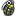•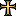••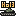•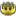•••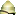•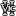••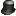I've done some tweaking here and there and unless someone disagrees with my changes I'll submit this one as per the rules.

Nice compilation. Small suggestion would be to up Königsmarck's movement to 5 to represent him better. Even if you'd have to reduce fire or shock to not make him too powerful.

#### unmerged(80927)

##### Corporal
I changed his maneuver value from 5 to 4 because I was under the impression that the abilities of the generals in EU2 only represent their tactical abilities. Königsmarck had skillful marches, outmaneuvering of enemies (like some imperial general in Saxony, I think) etc. Even during battle he was good but I don't know of any battles of him except Prague in 1648, where he did show himself a fairly good general even in the battlefield. I think he was quite helpful to Torstensson as well.

In any case, that's same reason why I reduced Sandels' maneuver value even further to 4. EU2 doesn't represent generals campaigning abilities. Some of the developers seem to think that, though. For example the English general Moore has a maneuver rating of 5, probably because of his retreat in the face of Soult, even though I think he only deserves a 4 or 3.

#### Norrefeldt

##### Porphyrogenitus
All great comments Legu! The leader file of Sweden needed som revision.

Did Stålhandske have any independent commands? I vaguely recall discussing this fellow before, and that he was removed because he didn't.
For Adlercreutz, I think a lower rank than his final 1 could be used, as half his time as a leader was with lower rank. If we always use the final rank, a very small portion of the scale will be used.
Herman Wrangel had an independent command when he won at Gurzno 1629. I think that is merit enough.

When the first major overhaul of leaders was done in AGCEEP, for all Italian states, the standard of not including more than the family names, unless there is a risk to mix up several historical persons. Not that it was fully implemented for all countries, or that it is strictly necessary.

#### unmerged(80927)

##### Corporal
On the other hand he had no actual naval commands. At least I think so.

For Adlercreutz, I think a lower rank than his final 1 could be used, as half his time as a leader was with lower rank. If we always use the final rank, a very small portion of the scale will be used.

Are we looking at the same post. I removed him for #47.

Why? Well, he only won battles when he outnumbered the Russians and when he had a good subordinate with him, like Döbeln. In those victories he was also way too cautious and failed to pursue them when they were defeated. He also lost the important battle, Oravais.

When the first major overhaul of leaders was done in AGCEEP, for all Italian states, the standard of not including more than the family names, unless there is a risk to mix up several historical persons. Not that it was fully implemented for all countries, or that it is strictly necessary.

Well, I don't think first names are very important but then again, unless those names are long, they don't do any harm, do they?. They're important for those Horns, of course.

I've changed Cronstedt's and Döbeln's rank as per your suggestion for Adlercreutz. Also removed the unnecessarily long names for those explorers. Bernhard's rank was corrected, as it should have been 1 like his other Swedish incarnation.

Code:
``````historicalleader = {
id = { type = 6 id = 0131847 }
category = general
name = "Bernhard"
startdate = {
day = 5
month = september
year = 1635
}
deathdate = {
day = 19
month = july
year = 1639
}
rank = [COLOR="Red"]0[/COLOR] [COLOR="Yellow"]1[/COLOR]
movement = 3
fire = 4
shock = 4
siege = 1
dormant = yes
remark = "if France refuses to hire him..."
}
id = { type = 6 id = 0131855 }
category = explorer
name = "[COLOR="Red"]Anders Nilsson[/COLOR] Krober"
startdate = {
year = 1637
}
deathdate = {
year = 1638
}
rank = 7
movement = 4
fire = 2
shock = 2
dormant = no
remark = "Led first expedition to New Sweden"
}
id = { type = 6 id = 0131856 }
category = explorer
name = "[COLOR="Red"]Cornelis van[/COLOR] Vliet"
startdate = {
year = 1639
}
deathdate = {
year = 1641
}
rank = 7
movement = 4
fire = 2
shock = 2
siege = 0
dormant = no
remark = "Set out to find location of West Indies"
}
id = { type = 6 id = 0131844 }
name = "Cronstedt"
startdate = {
year = 1789
}
deathdate = {
day = 2
month = december
year = 1801
}
rank = [COLOR="Red"]2[/COLOR] [COLOR="Yellow"]3[/COLOR]
movement = 3
fire = 4
shock = 2
siege = 0
dormant = no
}
id = { type = 6 id = 0131849 }
category = general
name = "Döbeln"
startdate = {
month = august
year = 1808
}
deathdate = {
day = 15
month = february
year = 1820
}
rank = [COLOR="Red"]2[/COLOR] [COLOR="Yellow"]3[/COLOR]
movement = 4
fire = 3
shock = 4
siege = 0
dormant = no
}``````

Herman Wrangel had an independent command when he won at Gurzno 1629. I think that is merit enough.

Ok

Code:
``````[COLOR="Yellow"]historicalleader = {
id = { type = 6 id = 0131819 }
category = general
name = "Herman Wrangel"
startdate = {
day = 7
month = august
year = 1627
}
deathdate = {
year = 1631
}
rank = 1
movement = 3
fire = 4
shock = 3
siege = 0
dormant = no
}[/COLOR]``````

I agree he merits being a leader.

#### Norrefeldt

##### Porphyrogenitus
Fine. I don't feel strongly about how the names are configured either, as long as they fit into the box. I think there once was a scprit tip for that, that I have forgotten.